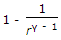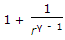# Mechanical Engineering - Thermodynamics

### Exercise :: Thermodynamics - Section 6

6.

The air standard efficiency of an Otto cycle is given by (where r = Compression ratio, and γ = Ratio of specific heats)

 A. 1 - rγ - 1 B. 1 + rγ - 1 C.D.Explanation:

No answer description available for this question. Let us discuss.

7.

In an ideal gas turbine plant, it is assumed that the compression and expansion processes are

 A. isothermal B. isentropic C. polytropic D. none of these

Explanation:

No answer description available for this question. Let us discuss.

8.

When gas is heated at constant pressure, the heat supplied is utilised in

 A. increasing the internal energy of gas B. doing some external work C. increasing the internal energy of gas and also for doing some external work D. none of the above

Explanation:

No answer description available for this question. Let us discuss.

9.

The amount of heat required to raise the temperature of 1 kg of water through one Kelvin is called

 A. specific heat at constant volume B. specific heat at constant pressure C. kilo-Joule D. none of these

Explanation:

No answer description available for this question. Let us discuss.

10.

When wood is heated with a limited supply of air to a temperature not less than 280°C, the resulting fuel is

 A. coke B. wood charcoal C. bituminous coal D. briquetted coal